# Dewey Decimal Classification as a Graph¶

We look at the DDC, from a graph theoretic approach. So each

• classification is a node
• relations between classifications - like hiearchy or see also, are edges
• Then the distance beteween two nodes, are a classifications relatedness

Dewey is often thought of as a hierarchy of relations, but in fact it's a more complex graph. Consider at least two more relations that can exist between classifications beyond being hierarchically related: "see also", and "class elsewhere".

Applications. Besides being a curiosty, we can use this to find some unexpected results.

Consider a Wikipedia Category, it can contain many Wikipedia articles, each of which has zero or citations of books. Some of those book citations include the ISBN, which is precisely what the OCLC Classify API is hungry for. In return for feeding it an ISBN, it will give you the Dewey Classification most popularly associated with the ISBN based on library data from WorldCat. That means we can arrive at a set of classifications associated with the category. From this set, it would be intructive if we could some how average them into one representative classification. In Graph Theory terms, this is known as finding the center, and there are several ways to approach this problem. The method we employ here is the betweenness centrality. That is, we calculate the weighted shortest paths between all the classifications associated with the category, and crown the nodes that we most touched in all the shortest paths. This is rather obsscure, so lets find out if it works on some examples.

So it seems that our method is roughly what we expect for German Military History, and Cooking, and Feminism. Namely pages in categories cite books whose classifications are similar to their own category topic. Maybe this is not surprising because sort of ciruclarly these pages are categorized because they rolve around a topic. But since our model seems relatively calibrated, let's try it on something less thematic. How about randomly choosing 500 of Wikipedia's Featured Articles.

In :
import xml.etree.ElementTree as ET
from collections import defaultdict
import networkx as nx
import matplotlib.pyplot as plt
import pyddc
import pymarc
import operator
import math
import json
import urllib2
import pywikibot
from pywikibot import pagegenerators as pg
import mwparserfromhell as pfh
%pylab inline

VERBOSE:pywiki:Starting 1 threads...

Populating the interactive namespace from numpy and matplotlib

In :
completedb = pymarc.parse_xml_to_array(open('CLASS_23eng_marc_webdewey_20131020.xml'))


Our rules:

• only if leader byte 8 is 'a'
• exclude spans, recrods that have eithe \$c or \$y in 153
• 253 ind 0 \$a "see reference" • 253 ind 2 \$a "class elsewhere"
• may be multiple 253s
• 153 \$a to$e is "notational hiearchy"

Later on tables:

• check for 008 6th byte is 'b' for table and 153 \$z exists • in 765 \$z is table num, \$s through \$t, relation will be "built from"
In :
graphs = defaultdict(nx.DiGraph)

f = defaultdict(int)

fcount = defaultdict(int)
for record in completedb:
class_num = None
table_num = None
tabl_class_num = None

for field in record.fields:

#conditon 153
if field.tag == '153':
y_z_subfields = field.get_subfields('y','z')

#nontables
if not y_z_subfields:
a_subfields = field.get_subfields('a')
a = a_subfields
class_num = a
#checking to see if we have notational hiearchy:
e_subfields = field.get_subfields('e')
if e_subfields:
#tables
'''
else:
z = field.get_subfields('z')
y = field.get_subfields('y')

if y and not z:
f['a']+=1
#print field
if z and not y:
f['b']+=1
print unicode(field)
'''

#condition 253
if field.tag == '253':

if field.indicator1 == '0':
y_z_subfields = field.get_subfields('y','z')
if not y_z_subfields:
a_subfields = field.get_subfields('a')
if a_subfields:
if class_num:

if field.indicator1 == '2':
y_z_subfields = field.get_subfields('y','z')
if not y_z_subfields:
a_subfields = field.get_subfields('a')
if a_subfields:
if class_num:
#this is if we want these relations to be symmetric

if field.tag == '765':

z = field.get_subfields('z')
s = field.get_subfields('s')

if z and s:
if len(z) == len(s):
if len(z) == 1:
if class_num:

In :
G = graphs['main']


## Weighting the Graph¶

Since we are looking at many different types of connections, if we want to be able to make a numeric comparison we can assign each relation a weight.

In :
weights_dict = {'notational_hiearchy': 0.5,
'see_reference':1,
'class_elsewhere':2}

In :
def update_weights(G, weights_dict):
W = nx.DiGraph()

for m in G.nodes():
for n in G[m].iterkeys():
relation = G[m][n]['rel']
weight = weights_dict[relation]

return W

In :
W = update_weights(G, weights_dict)

In :
def weighted_shortest_path(W, a, b):
path = nx.shortest_path(W, a, b, weight='rel')
return path
'''
total_length = 0

try:
except IndexError:
break

#print link_from, "- relation: ", relation, " weight: ", link_weight, " - ", link_to, " | " ,
'''

In :
weighted_shortest_path(W, '394.1', '341.33')

Out:
['394.1', u'394', u'395', u'327.2', u'341.33']
In :
nx.shortest_path_length(W, '394.1', '341.33', weight='rel')

Out:
5.5
In :
def weighted_length(G, path, weights_dict, print_path=False):
"""we can use this to calculate the path lenght, or just print it"""
total_length = 0

try:
except IndexError:
break

if print_path:
print link_from, "- relation: ", relation, " weight: ", link_weight, " - ", link_to, " | " ,

if not print_path:

In :
def multiple_betweenness(W, node_list):
between_count = defaultdict(int)
for a in node_list:
for b in node_list:
if a != b:
try:
path = weighted_shortest_path(W, a, b)
except nx.NetworkXNoPath:
continue
for node in path:
between_count[node] += 1
sorted_beteween_nodes = sorted(between_count.iteritems(), key=operator.itemgetter(1))
return sorted_beteween_nodes

In :
def node_in_G(G, node):
#recursively check and remove
if not node:
return False
try:
G[node]
return node
except:
nod = node[:-1]
node_in_G(G, nod)


### Results¶

Thus for the Category:English literature books. 153.6 is the class that is most inbetween

## Classify API¶

In :
def get_ddc_from_isbn(isbn):
base_path = 'http://classify.oclc.org/classify2/Classify?'
isbn_path = 'isbn='+str(isbn)
full_path = base_path+isbn_path+'&summary=true'

try:
req = urllib2.Request(url=full_path)
f = urllib2.urlopen(req)
root = ET.fromstring(xml_response)
for child in root:
if child.tag == '{http://classify.oclc.org}recommendations':
for rec in child:
if rec.tag == '{http://classify.oclc.org}ddc':
for pop in rec:
if pop.tag == '{http://classify.oclc.org}mostPopular':
try:
return pop.attrib['nsfa']
except:
return pop.attrib['sfa']
except:
print isbn_path

In :
def get_ddcs_from_categorgy(category_name):
cat = pywikibot.Category(pywikibot.Site('en', 'wikipedia'), category_name)
articles = list(cat.articles(total=None))
return get_ddcs_from_list(articles)

In :
def get_ddcs_from_list(articles):

isbns = []

for article in articles:
try:
wikitext = article.get()
except pywikibot.IsRedirectPage:
redir_page = article.getRedirectTarget()
wikitext = redir_page.get()
wikicode = pfh.parse(wikitext)
templates = wikicode.filter_templates()
for template in templates:
for param in template.params:
if param.name.lower().startswith('isbn'):
#print param.name, param.value
isbns.append(param.value)

isbn_set = set()
str_isbns = map(str, isbns)
for isbn in str_isbns:
justnums = filter(lambda x: x in '1234567890Xx', isbn)
if len(justnums) in [10,13]:

ddcs = map(get_ddc_from_isbn, list(isbn_set))
return ddcs

In :
def most_between_gen(articles):
potential_ddcs = get_ddcs_from_list(articles)
valid_ddcs = filter(lambda a: a,map(lambda ddc: node_in_G(W, ddc), potential_ddcs))
multi_between = multiple_betweenness(W, valid_ddcs)
top_10 = multi_between[-10:]
for top in top_10:
print top, ' ', top, ' ', search_caption(top)

In :
def most_between_category(category_name):
potential_ddcs = get_ddcs_from_categorgy(category_name)
valid_ddcs = filter(lambda a: a,map(lambda ddc: node_in_G(W, ddc), potential_ddcs))
multi_between = multiple_betweenness(W, valid_ddcs)
top_10 = multi_between[-10:]
for top in top_10:
print top, ' ', top, ' ', search_caption(top)

In [ ]:
most_between_category('Military_history_of_Germany')

In :
most_between_category('Cooking_techniques')

VERBOSE:pywiki:Found 1 wikipedia:en processes running, including this one.

100   641.58   [u'Cooking with specific fuels, appliances, utensils']
102   641.59   [u'Cooking characteristic of specific geographic environments, ethnic cooking']
108   641.302   [u'Health foods']
180   641.82   [u'Main dishes']
246   641.8   []
259   613.2   [u'Dietetics']
266   641.3   [u'Food']
270   641.5   []
276   641   [u'Ceuta and Melilla']

In :
cat = pywikibot.Category(pywikibot.Site('en', 'wikipedia'), 'Featured_articles')
articles = list(cat.articles(total=None))

isbns = []

for article in articles:
try:
wikitext = article.get()
except pywikibot.IsRedirectPage:
redir_page = article.getRedirectTarget()
wikitext = redir_page.get()
wikicode = pfh.parse(wikitext)
templates = wikicode.filter_templates()
for template in templates:
for param in template.params:
if param.name.lower().startswith('isbn'):
#print param.name, param.value
isbns.append(param.value)

VERBOSE:pywiki:Found 1 wikipedia:en processes running, including this one.
VERBOSE:pywiki:Found 1 wikipedia:en processes running, including this one.
VERBOSE:pywiki:Found 1 wikipedia:en processes running, including this one.
VERBOSE:pywiki:Found 2 wikipedia:en processes running, including this one.
VERBOSE:pywiki:Found 2 wikipedia:en processes running, including this one.
VERBOSE:pywiki:Found 1 wikipedia:en processes running, including this one.
VERBOSE:pywiki:Found 1 wikipedia:en processes running, including this one.
VERBOSE:pywiki:Found 1 wikipedia:en processes running, including this one.
VERBOSE:pywiki:Found 1 wikipedia:en processes running, including this one.
ERROR: Traceback (most recent call last):
File "/home/notconfusing/workspace/pwb-core/pywikibot/data/api.py", line 291, in submit
body=paramstring)
File "/home/notconfusing/workspace/pwb-core/pywikibot/comms/http.py", line 141, in request
raise request.data
ServerNotFoundError: Unable to find the server at en.wikipedia.org

ERROR:pywiki:Traceback (most recent call last):
File "/home/notconfusing/workspace/pwb-core/pywikibot/data/api.py", line 291, in submit
body=paramstring)
File "/home/notconfusing/workspace/pwb-core/pywikibot/comms/http.py", line 141, in request
raise request.data
ServerNotFoundError: Unable to find the server at en.wikipedia.org

VERBOSE:pywiki:/w/api.php, maxlag=5&format=json&rvprop=ids%7Cflags%7Ctimestamp%7Cuser%7Ccomment%7Ccontent&prop=info%7Crevisions&titles=Wonder+Stories&meta=userinfo&indexpageids=&action=query&uiprop=blockinfo%7Chasmsg
WARNING: Waiting 5 seconds before retrying.
WARNING:pywiki:Waiting 5 seconds before retrying.

In :
ddcs?

In :
isbn_set = set()
str_isbns = map(str, isbns)
for isbn in str_isbns:
justnums = filter(lambda x: x in '1234567890Xx', isbn)
if len(justnums) in [10,13]:

ddcs = map(get_ddc_from_isbn, list(isbn_set))

isbn=9781860649318
isbn=9780760311233
isbn=0405140991
isbn=0521417864
isbn=0071116583
isbn=9780980346695
isbn=0811727777
isbn=9781402063121
isbn=9780786425921
isbn=9780820330716
isbn=1933648716
isbn=0198584075
isbn=0786403950
isbn=9780670085729
isbn=9780415192767
isbn=9810229402
isbn=0839535945
isbn=0027885208
isbn=184681197X
isbn=0394425952
isbn=0876616414
isbn=0060176172
isbn=9780803277946
isbn=0521564387
isbn=0719557658
isbn=0849321727
isbn=0195126335
isbn=1905326173
isbn=1845830377
isbn=0306808544
isbn=9780292701533
isbn=0275994929
isbn=0755313925
isbn=9780521033732
isbn=0521224799
isbn=0824809599
isbn=9789185509362
isbn=9780760308929
isbn=0874804477
isbn=0028645995
isbn=9780345491480
isbn=0968910807
isbn=9780643066359
isbn=013843557X
isbn=9780814713174
isbn=3613014599
isbn=0307377229
isbn=0719035422
isbn=9780306819100

In :
json.dump(ddcs, open('featuredddcs,json','w'))

In :
ddcs = json.load(open('featureddccs.json','r'))

In :
len(ddcs)

Out:
17100
In :
uniqddcs = list(set(ddcs))
valid_ddcs = filter(lambda a: a,map(lambda ddc: node_in_G(W, ddc), uniqddcs))
json.dump(valid_ddcs, open('valid_ddcs,json','w'))

In :
smalluniqddcs = uniqddcs[-500:]

In :
%%timeit -n1 -r1
valid_ddcs = filter(lambda a: a,map(lambda ddc: node_in_G(W, ddc), smalluniqddcs))
multi_between = multiple_betweenness(W, valid_ddcs)
top_10 = multi_between[-10:]
for top in top_10:
print top, ' ', top, ' ', search_caption(top)

518   338.9   Economic development and growth
533   616.8   []
None
536   338   []
None
620   900   []
None
630   97   North American native languages
630   971   Inuit, Yupik, Aleut languages
1014   800   []
None
1145   361   []
None
1362   940   Military operations and units
1461   930   []
None
1 loops, best of 1: 25min 13s per loop

In :
most_between_category('Featured_articles')

VERBOSE:pywiki:Found 1 wikipedia:en processes running, including this one.
VERBOSE:pywiki:Found 1 wikipedia:en processes running, including this one.
VERBOSE:pywiki:Found 1 wikipedia:en processes running, including this one.
VERBOSE:pywiki:Found 1 wikipedia:en processes running, including this one.
VERBOSE:pywiki:Found 1 wikipedia:en processes running, including this one.
VERBOSE:pywiki:Found 1 wikipedia:en processes running, including this one.
VERBOSE:pywiki:Found 1 wikipedia:en processes running, including this one.
VERBOSE:pywiki:Found 1 wikipedia:en processes running, including this one.

---------------------------------------------------------------------------
URLError                                  Traceback (most recent call last)
<ipython-input-97-d88e6e75f36f> in <module>()
----> 1 most_between_category('Featured_articles')

<ipython-input-58-82e1fc0d0725> in most_between_category(category_name)
1 def most_between_category(category_name):
----> 2     potential_ddcs = get_ddcs_from_categorgy(category_name)
3     valid_ddcs = filter(lambda a: a,map(lambda ddc: node_in_G(W, ddc), potential_ddcs))
4     multi_between = multiple_betweenness(W, valid_ddcs)
5     top_10 = multi_between[-10:]

<ipython-input-96-c39a6a07a9bc> in get_ddcs_from_categorgy(category_name)
2     cat = pywikibot.Category(pywikibot.Site('en', 'wikipedia'), category_name)
3     articles = list(cat.articles(total=None))
----> 4     return get_ddcs_from_list(articles)

<ipython-input-82-e704291a5063> in get_ddcs_from_list(articles)
25
---> 26     ddcs = map(get_ddc_from_isbn, list(isbn_set))
27     return ddcs

<ipython-input-20-052a8ac7c399> in get_ddc_from_isbn(isbn)
5
6     req = urllib2.Request(url=full_path)
----> 7     f = urllib2.urlopen(req)
9     root = ET.fromstring(xml_response)

/usr/lib/python2.7/urllib2.pyc in urlopen(url, data, timeout)
125     if _opener is None:
126         _opener = build_opener()
--> 127     return _opener.open(url, data, timeout)
128
129 def install_opener(opener):

/usr/lib/python2.7/urllib2.pyc in open(self, fullurl, data, timeout)
402             req = meth(req)
403
--> 404         response = self._open(req, data)
405
406         # post-process response

/usr/lib/python2.7/urllib2.pyc in _open(self, req, data)
420         protocol = req.get_type()
421         result = self._call_chain(self.handle_open, protocol, protocol +
--> 422                                   '_open', req)
423         if result:
424             return result

/usr/lib/python2.7/urllib2.pyc in _call_chain(self, chain, kind, meth_name, *args)
380             func = getattr(handler, meth_name)
381
--> 382             result = func(*args)
383             if result is not None:
384                 return result

/usr/lib/python2.7/urllib2.pyc in http_open(self, req)
1212
1213     def http_open(self, req):
-> 1214         return self.do_open(httplib.HTTPConnection, req)
1215
1216     http_request = AbstractHTTPHandler.do_request_

/usr/lib/python2.7/urllib2.pyc in do_open(self, http_class, req)
1182         except socket.error, err: # XXX what error?
1183             h.close()
-> 1184             raise URLError(err)
1185         else:
1186             try:

URLError: <urlopen error [Errno -2] Name or service not known>
In :
most_between_category('Feminism')

VERBOSE:pywiki:Found 1 wikipedia:en processes running, including this one.

309   305.4209   []
None
313   305.3   People by gender or sex
355   305.909   Generalists
359   306.3   Economic institutions
369   306.36   Systems of labor
595   305.4   Women
602   305   []
None
609   305.9   People by occupation and miscellaneous social statuses; people with disabilities and illnesses, gifted people
661   305.43   Women by occupation
1363   305.42   Social role and status of women

In :
maxs_contribs = list(pg.UserContributionsGenerator(username='Maximilianklein', namespaces=0))

VERBOSE:pywiki:Found 1 wikipedia:en processes running, including this one.

In :
len(maxs_contribs)

Out:
307
In :
most_between_gen(maxs_contribs)

1414   782.4   Secular forms
1414   782.1   General principles and musical forms
1515   782.42166092   []
None
1606   782.42   Songs
1696   361   []
None
1784   910.45   Ocean travel and seafaring adventures
1900   302.2   Communication
1959   302.23   Media (Means of communication)
2421   800   []
None
3091   930   []
None

In :
def search_caption(s_class):

for record in completedb:
class_num = None
table_num = None
tabl_class_num = None

for field in record.fields:

#conditon 153
if field.tag == '153':
a_subfields = field.get_subfields('a')
if a_subfields == s_class:
h = field.get_subfields('h')
j = field.get_subfields('j')
k = field.get_subfields('k')
try:
return j
except:
print j
return None


## Visualisations¶

In :
def neighbors_n(G, root, n):
E = nx.DiGraph()

def n_tree(tree, n_remain):
neighbors_dict = G[tree]

for neighbor, relations in neighbors_dict.iteritems():

#you can use this map if you want to retain functional purity
#map(lambda neigh_rel: E.add_edge(tree, neigh_rel, rel=neigh_rel['rel']), neighbors_dict.iteritems() )

neighbors = list(neighbors_dict.iterkeys())
n_forest(neighbors, n_remain= (n_remain - 1))

def n_forest(forest, n_remain):
if n_remain <= 0:
return
else:
map(lambda tree: n_tree(tree, n_remain=n_remain), forest)

n_forest( [root] , n)

return E

def draw_n_hops(G, root, n, figsizex=20, figsizey=20):
E = neighbors_n(G, root, n)
edge_rels = map(lambda edge_tup: edge_tup['rel'], E.edges(data=True) )
edge_colors = map(lambda edge: {'notational_hiearchy':'red', 'see_reference':'green', 'class_elsewhere':'blue'}[edge], edge_rels)

max_node_size = max( map(lambda node: nx.degree(E, node), E.nodes()))

node_lognorms = map(lambda node: (math.log(nx.degree(E, node)) / math.log(max_node_size) ) , E.nodes() )
node_sizes = map(lambda norm: norm * 1500, node_lognorms)

node_colors= node_lognorms

pos=nx.graphviz_layout(E,prog="twopi",root=root)

plt.figure(1,figsize=(figsizex,figsizey))
nx.draw(E, pos=pos, node_size=node_sizes, node_color=node_colors, edge_color=edge_colors)



Below is a visualization of all the classifications $n$-relations away from the classification "394.1" for $n \in \{2,3,4,5,6,7,10\}$.

• Colour: Red links are notational hierarchicy Blue are class elsewhere *Green are "see" references
• The node size and "hotness" are the number of incoming relations (the degree of the node)
In :
draw_n_hops(G, '394.1', 2, figsizex=12, figsizey=12)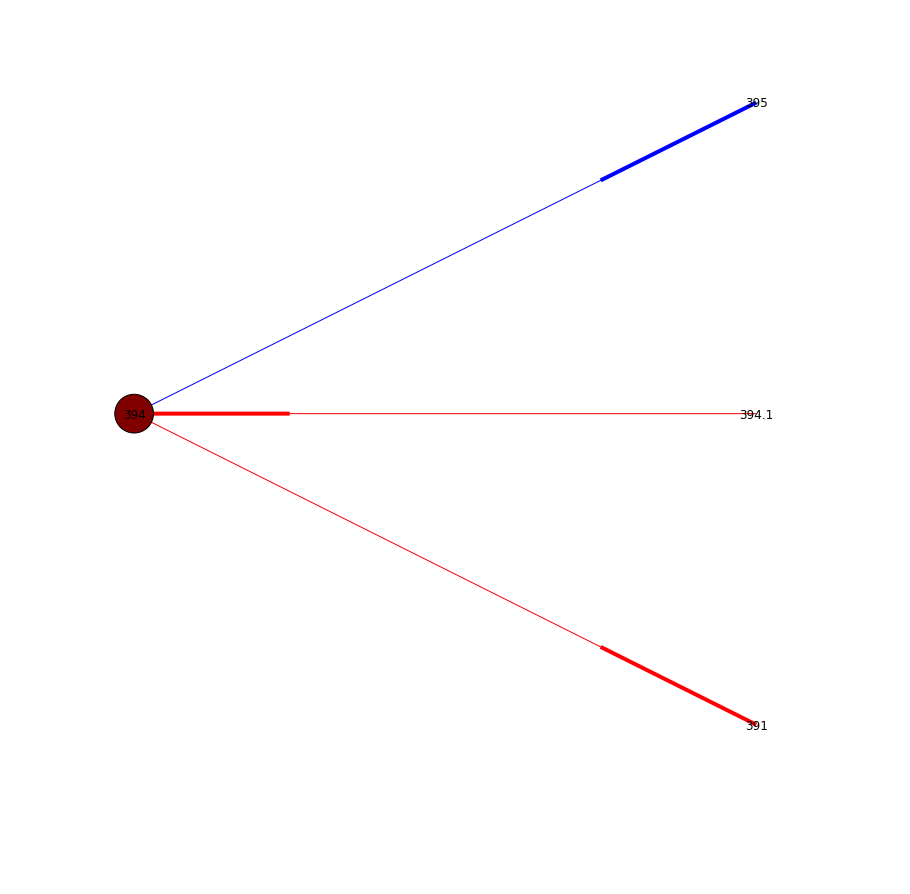In :
draw_n_hops(G, '394.1', 3, figsizex=12, figsizey=12)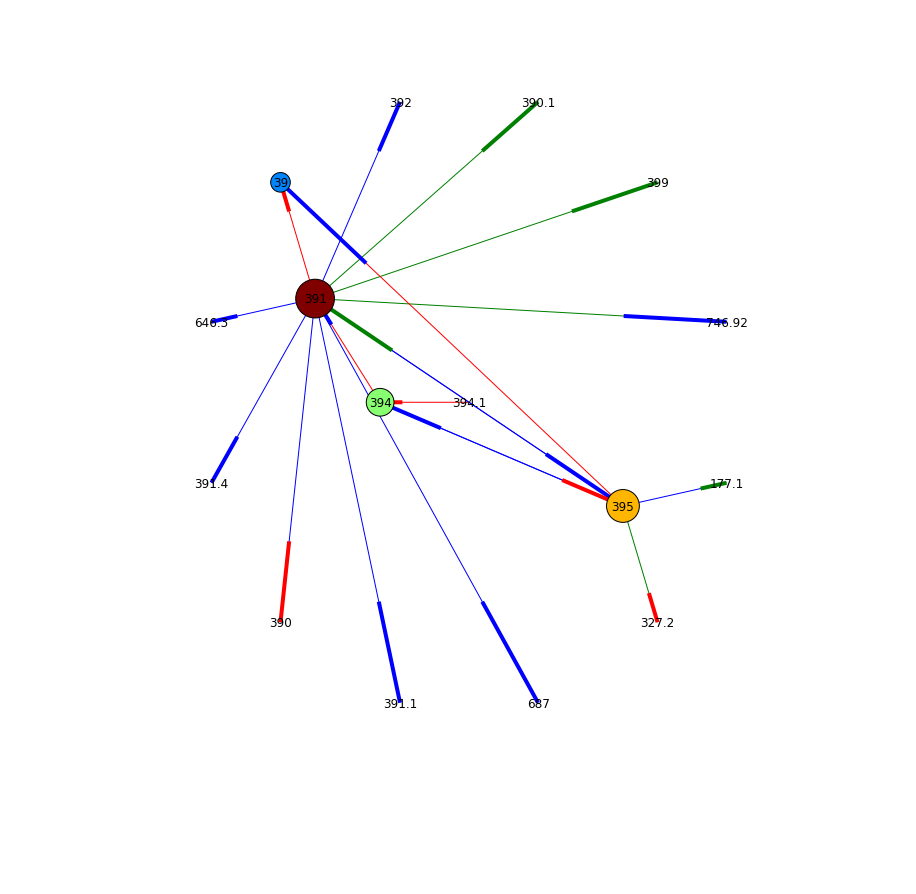In :
draw_n_hops(G, '394.1', 4, figsizex=12, figsizey=12)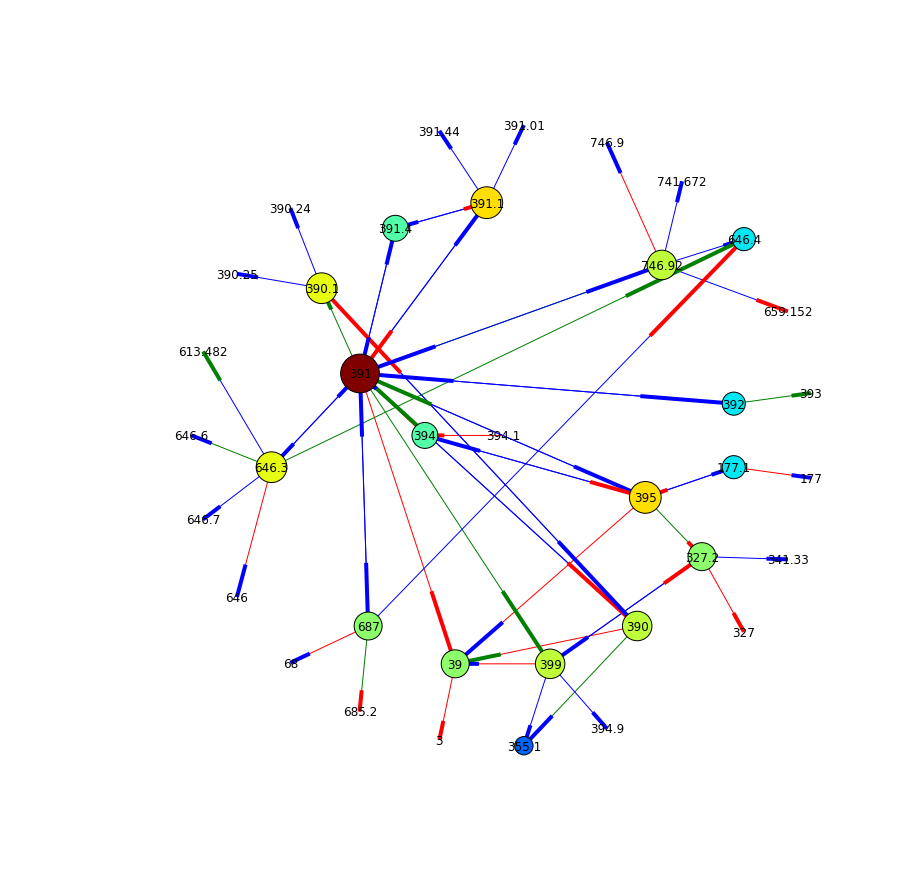In :
draw_n_hops(G, '394.1', 5, figsizex=12, figsizey=12)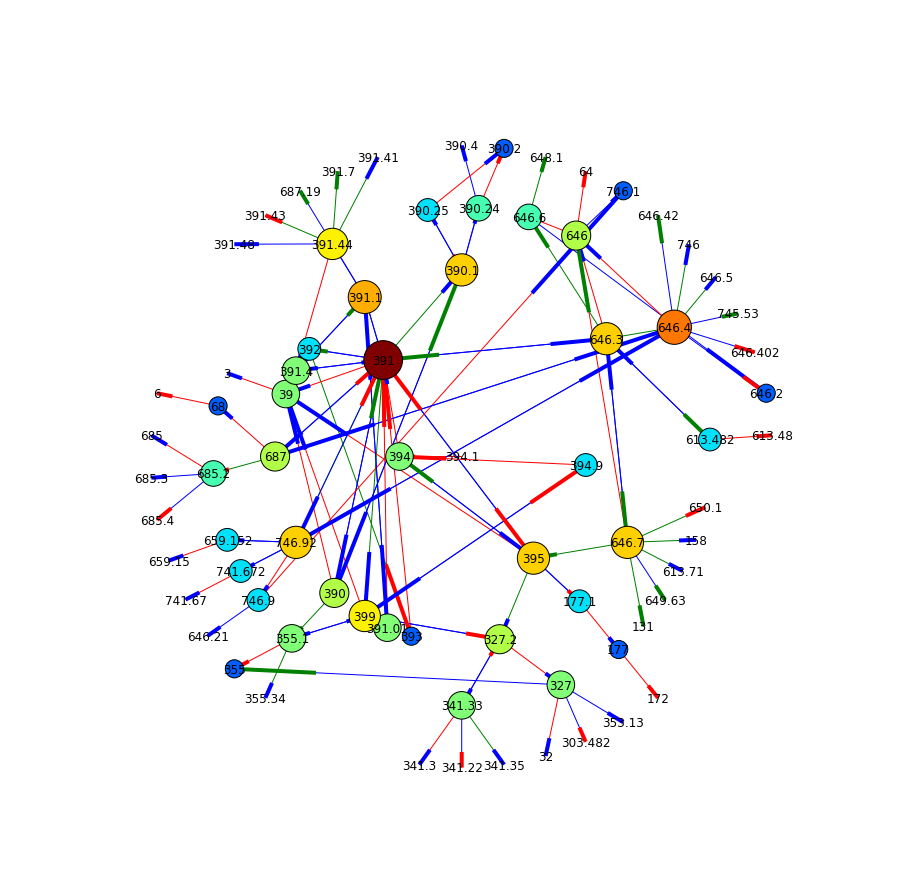In :
draw_n_hops(G, '394.1', 6, figsizex=12, figsizey=12)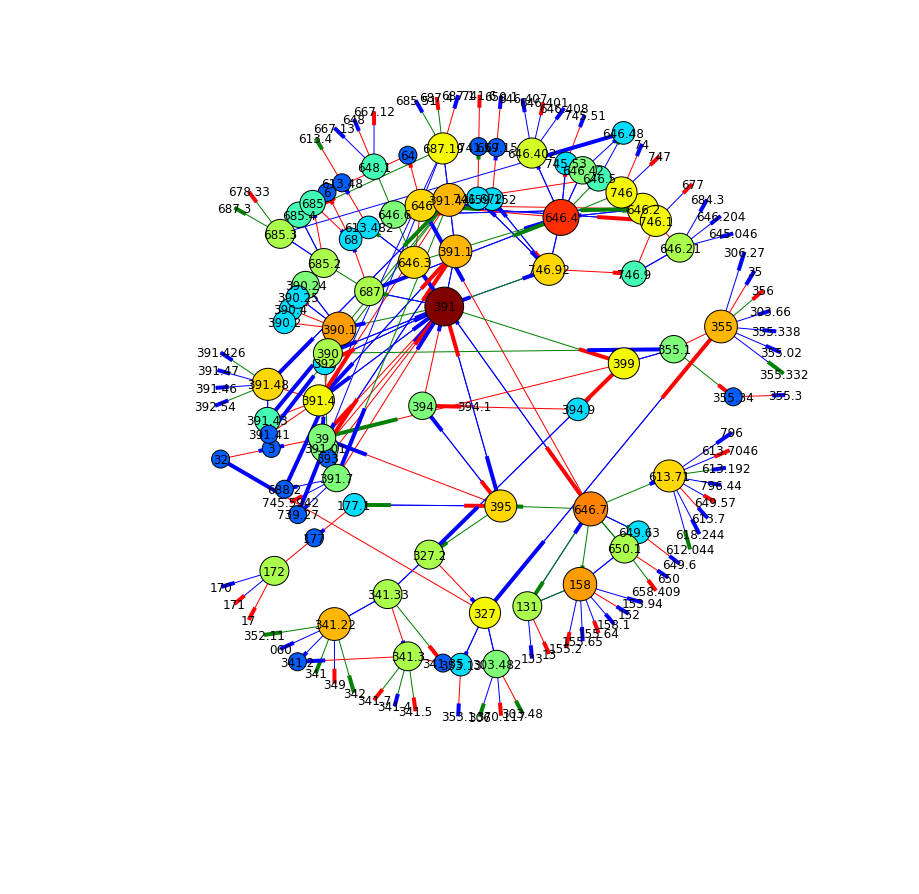In :
draw_n_hops(G, '394.1', 7, figsizex=12, figsizey=12)